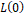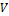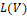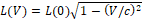### Consistency of the Ether Theory with the Time Concept, the Mass Concept and the Special Relativity

•  David Zareski

#### Abstract

We demonstrate that it is by considering the existence of an “ether” that one can define the notions of time, velocity and mass, and prove that Einstein’s second principle implies the existence of this ether since the light propagates independently of its emitter velocity. Then, that the particle velocity is a group velocity different from the phase velocity when the particle is massive. One interprets then the Morley-Michelson experiment in the frame of the ether.

Let be L0a fixed distance between two points immobile in a long vehicle in the ether, and let a photon that, when this vehicle is immobile relatively to the ether, travels the distance L0in this vehicle. An observer immobile in the immobile vehicle sees that the distance traveled by the photon is also L0. But, if the vehicle moves, e.g., at the velocity Vrelatively to the ether, and the photon at the velocity calso relatively to the ether, then an observer immobile in the moving vehicle will find that the distance traveled by this photon is not L0but is L(V)defined by

L(V)=L(0)1-V/c2). We prove then that L(V)is not the new dimension of the vehicle, but is simply the distance travelled by the photon in this vehicle. That is, as demonstrated in our previous publications: the ether volumetric density is constant.

• Full Text:PDF
• DOI:10.5539/apr.v12n4p1This work is licensed under a Creative Commons Attribution 4.0 License.
• ISSN(Print): 1916-9639
• ISSN(Online): 1916-9647
• Started: 2009
• Frequency: bimonthly